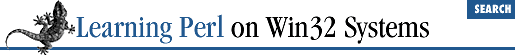home | O'Reilly's CD bookshelfs | FreeBSD | Linux | Cisco | Cisco Exam

### 3.2 Literal Representation

A list literal (the way you represent the value of a list within your program) consists of comma-separated values enclosed in parentheses. These values form the elements of the list. For example:

```(1,2,3)      # array of three values 1, 2, and 3
("fred",4.5) # two values, "fred" and 4.5```

The elements of a list are not necessarily constants - they can be expressions that will be evaluated newly each time the literal is used. For example:

```(\$a, 17)      # two values: the current value of \$a, and 17
(\$b+\$c,\$d+\$e) # two values```

The empty list (one of no elements) is represented by an empty pair of parentheses:

`() # the empty list (zero elements)`

An item of the list literal can include the list constructor function , indicated by two scalar values separated by two consecutive periods. This function creates a list of values starting at the left scalar value and continuing up through the right scalar value, incrementing by one at each value. For example:

```(1..5)       # same as (1, 2, 3, 4, 5)
(1.2..5.2)   # same as (1.2, 2.2, 3.2, 4.2, 5.2)
(2..6,10,12) # same as (2,3,4,5,6,10,12)
(\$a..\$b)     # range determined by current values of \$a and \$b```

Having the right scalar less than the left scalar results in an empty list; you can't count down by switching the order of the values. If the final value is not a whole number of steps above the initial value, the list stops just before the next value would have been outside the range:

`(1.3..6.1) # same as (1.3,2.3,3.3,4.3,5.3)`

List literals with lots of short text strings start to look pretty noisy with all the quotes and commas:

`@a = ("fred","barney","betty","wilma"); # ugh!`

Fortunately, Perl has a shortcut: the "quote-word" syntax, which creates a list from the nonwhitespace parts between the parentheses:

```@a = qw(fred barney betty wilma); # better!
@a = qw(
fred
barney
betty
wilma
);                                # same thing```

One use of a list literal is as arguments to the ``` print()``` function introduced earlier. Elements of the list are printed out without any intervening whitespace:

`print("The answer is ",\$a,"\n"); # three element literal array`

This statement prints "``` The answer is``` " followed by a space, the value of ``` \$a``` , and a newline. Stay tuned for other uses for list literals.3.1 What Is a List or Array?3.3 Variables